##### Search### 1. SECTION I (50 Marks)

1.

Use logarithm tables to evaluate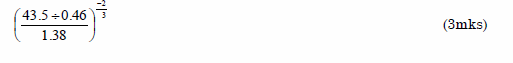3 marks

2.

A perpendicular line is drawn from a point (1,2) to the line 3y + 2x+1 = 0 . Find the
equation of the perpendicular line in the form y=mx+c (3mks)

3 marks

3.

Simplify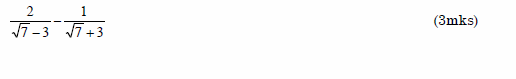3 marks

4.

Illustrate on a number line the solution of the following inequality (2mks)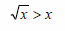2 marks

5.

A quantity y is partly constants and partly varies as x. When x=3 and y=1 and when
x=4 , y=10. Find y in terms of x, hence find y when x=6. (4mks)

4 marks

6.

The size of an interior angle of a rectangular polygon is 3x while its corresponding
exterior angle is (x-20)0. Find the number of sides of the polygon. (3mks)

3 marks

7.

a) Expand and simplify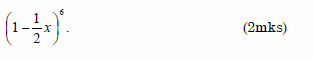b) Use the expansion up to the fourth term to find the value of (0.98)6 to four decimal
places. (2mks)

3 marks

8.

The image of the point (0,2), under an enlargement with scale factor 3 is (4,6). Find
the centre of enlargement. (3mks)

3 marks

9.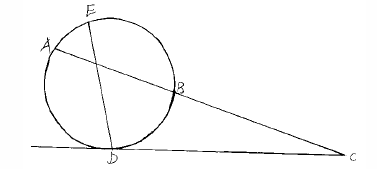In the figure above AB=5cm and CD=7cm. DC is the tangent to the circle. Find the
length of BC. (3mks)

3 marks

10.

Make r the subject of the formula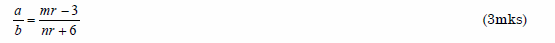3 marks

11.

A shopkeeper mixes sugar costing ksh.120 per kg with another type which costs
ksh.140 per kg. Find the ratio in which the two types should be mixed so that if a
kilogram of the mixture is sold at ksh.150, a profit of 20% is realized. (3mks)

3 marks

12.

Mr. Maina deposits ksh 60,000 in a bank which pays 12% interest per annum
compounded quarterly. Find the amount in the account after 3years. (3mks)

3 marks

13.

Find the centre and radius of a circle which is given by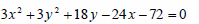(3mks)

3 marks

14.

The position vectors for points M and N are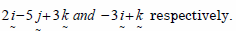Find
the length MN. (3mks)

3 marks

15.

What is the percentage error in using 0.33 in estimating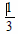(3mks)

3 marks

16.

Write down the inequalities represented by the region R. (4mks)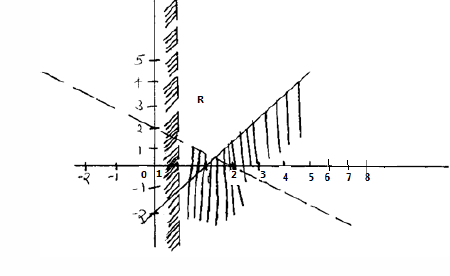4 marks

### 2. SECTION II (50 Marks)

17.

Income rates for income earned were charged as follows.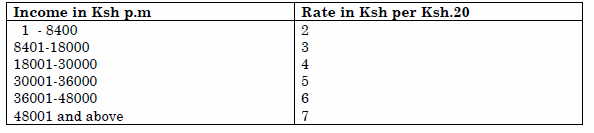Mrs. Lunani a civil servant earns a basic salary of Ksh.30,000. Her house allowance in ksh 12000 per month. Other allowances are transport ksh. 1300, and medical allowance of ksh 2500. She is entitled to a family relief of ksh 1240 per month. Calculate;
a) i) Her taxable income per month. (2mks)

ii) Net tax. (5mks)

NHIF Ksh 230
Service charge Ksh 100
Loan repayment Ksh 5000
Co-operatives shares Ksh 2000
Calculate her net salary (3mks)

10 marks

18.

The table below shows marks scored by 100 form four candidates in a mathematics
test.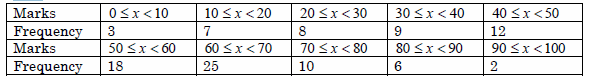a) Draw a cumulative frequency graph to illustrate the data. (4mks)

i) The median (1mk)

ii) Semi- interquartile range (3mks)

iii) The pass mark if 65% of students are to pass. (2mks)

10 marks

19.

An arithmetic progression has the first term a and common difference d.
a) Write down the third, ninth and twenty fifth terms of progression in terms of a and d. (3mks)

b) The arithmetic progression above is increasing and that the third, ninth and twenty fifth terms form the first three consecutive terms of a geometric progression. The sum of the seventh and twice the sixth terms of the arithmetic progression of 78.
Calculate:
i) The first term and common difference of the arithmetic progression. (5mks)

ii) The sum of the first nine term of the arithmetic progression. (2mks)

10 marks

20.

In the figure below E, is the mid-point of BC; AD:DC=3:2 and F is the meeting point of BD and AE.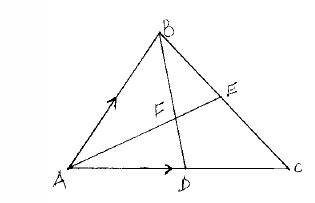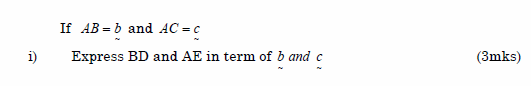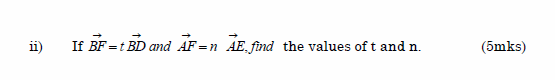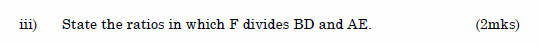10 marks

21.

A parallelogram has vertices A(0,0), B(-3,1), C(1,3) and D(4,2)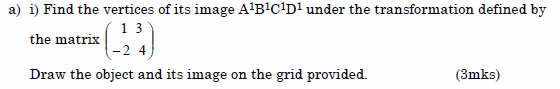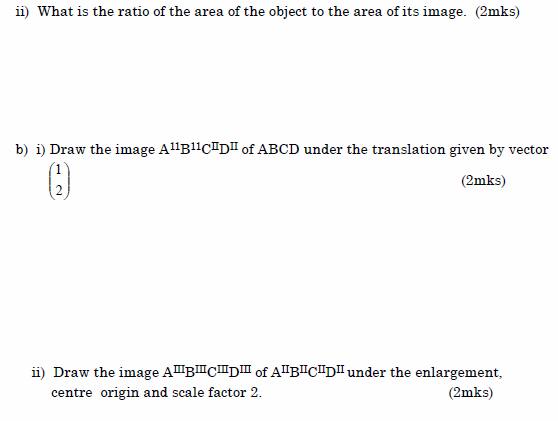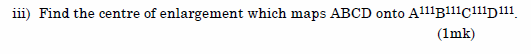10 marks

22.

A bag contains 5 green and 3 white marbles. Three marbles are drawn at random without replacement.
a) Determine the probability that:
i) More green marbles than white marbles are drawn. (4mks)

ii) At least one green marbles is drawn. (4mks)
iii) The marbles drawn are of the same colour. (2mks)

10 marks

23.

The figure below shows circles that intersect at points A and B. Point E is the centre of the smaller circle and lies on the circumference of the larger one.
FAC and FBD are straight lines.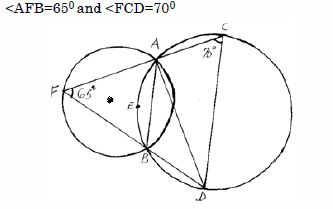Calculate:
a) The obtuse angle AEB (2mks)

Giving reasons find the value of:
b) Angle ACB (3mks)

d) Angle EBF (2mks)

10 marks

24.

a) Find the inverse of a matrix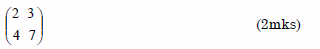b) Nasimiyu bought 2 chemistry books and 3 mathematical books at a total of ksh 2100, Wakachala bought 4 chemistry books and 7 mathematics books for a total of ksh 4700.
i) Form a matrix equation to represent the above information. (1mk)

ii) Use matrix method to find the price of a chemistry book and that of a mathematics book. (3mks)

c) Wesimikha High School bought 40 chemistry books and 50 mathematics books. A discount of 5% was allowed on each chemistry book whereas a discount of 8% was allowed on each mathematics book.
Calculate the percentage discount on the cost of all the books bought. (4mks)

10 marks

Back Top